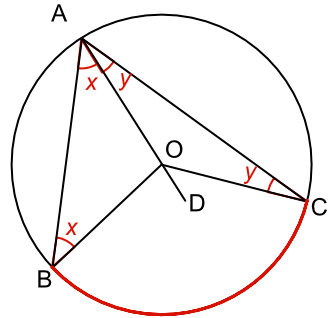Circle Theorems - Subtended Angles

## Circle Theorems - Subtended Angles

The angle at the centre of a circle is twice the angle at the circumference when both are subtended by the same arc.

Proof: an arc BC is drawn on the circumference of a circle. Draw a line from these points to the centre of the circle (lines BO and CO). Draw also a line from these points to a point on the circumference (lines BA and CA).

A line from point A is extended through the centre to point D to create two triangles.

Angle ∠BAO = angle ∠ABO = x (AO, BO and CO are radii; both triangles are isosceles).

The value of angle ∠BOD = 2x (∠AOB = 180 - 2x; and ∠BOD = 180 - (180 - ∠AOB))

Similarly for the other triangle, ∠COD = 2y

Angle ∠BAC = x + y

Angle ∠BOC = 2x + 2y = 2(x + y)

Therefore the angle at the centre of a circle is twice the angles at the circumference when subtended by the same arc.## Example 1

What is the size of the angle ∠BOC?The angle is twice the size of the angle ∠BAC (angle at the centre of a circle is twice the angle at the circumference when subtended by the same arc).

## Example 2

What is the size of the angle ∠BAC?Triangle BOC is an isosceles triangle (OB and OC are radii); therefore angle ∠OCB = 42º.

Angle ∠BOC = 180 - 42 - 42 = 96º

Angle subtended at the centre is twice the angle subtended at the circumference when on the same arc: 96 ÷ 2 = 48º